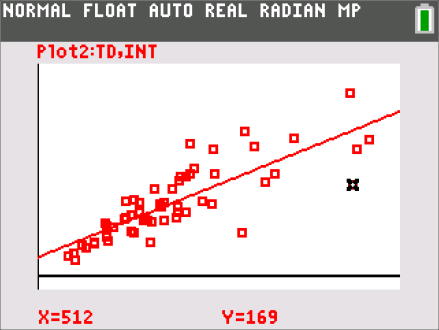••• ##### Device
•TI-84 Plus C Silver Edition
•TI-84 Plus CE
• ##### Software

TI Connect™ CE
TI-SmartView™ CE

# Mathematical Modeling: Modeling QB Passing Rates

by Texas Instruments#### Objectives

• Students will model a contextual situation mathematically and use the model to answer a question.
• Students will represent data on two quantitative variables on a scatter plot, and describe how the variables are related.
• Students will find and interpret linear equations to model relationships between two quantitative variables.
• Students will use proportional relationships to solve real-world and mathematical problems.